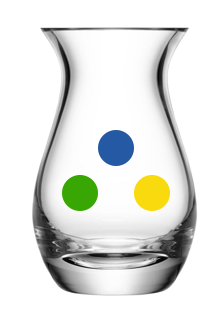# Multinomial Distribution

Probability Level 3An urn contains 1 blue, 1 green and 1 yellow ball. With replacement, we draw out a ball 7 times and record down the color seen. What is the probability that we see an equal number of blue and green balls?

The answer can be written as $\dfrac{a}{b}$ with $a$ and $b$ coprime positive integers. Submit $a+b$.

×